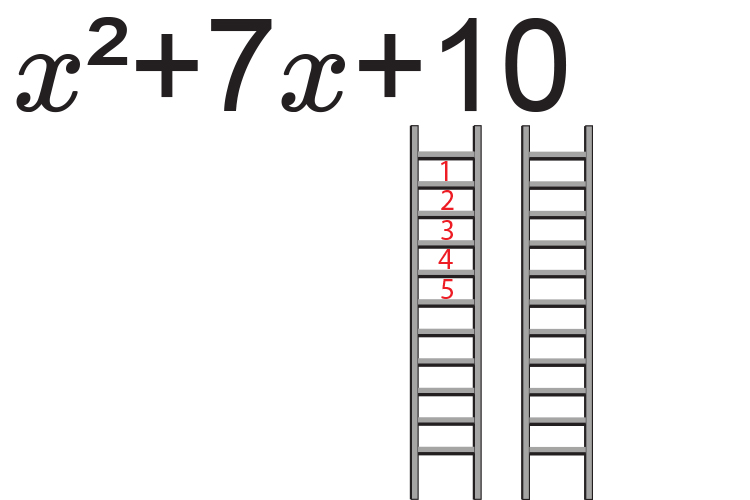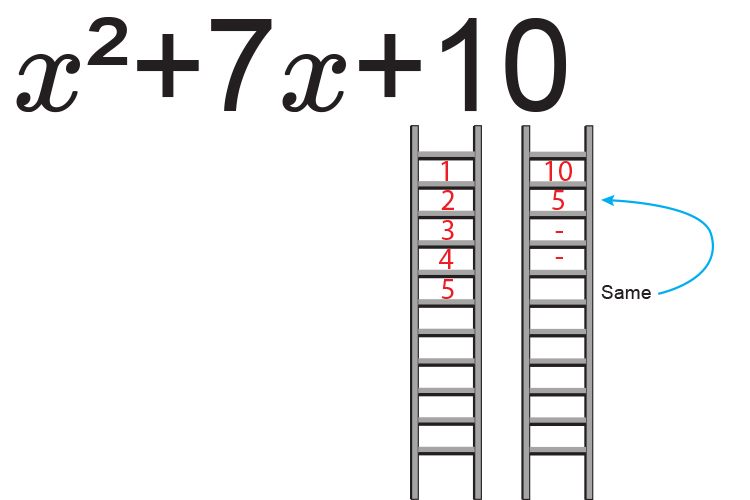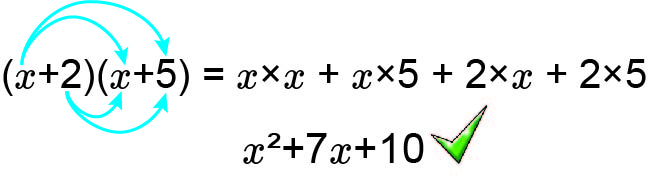# Factorising quadratics - (easy) Example 1

Factorise the quadratic x^2+7x+10Therefore the factors of 10 are:

1 and 10

2 and 5

Now ask yourself what factors of c  add to give b.

i.e. what factors of 10 equal 7.

5times2=10           5+2=7

Therefore:

x^2+7x+10

Can be written as:

(x+2)(x+5)

Check by multiplying out:The shock is that (x+2)(x+5)  also gives you the roots of x^2+7x+10=0

Separate the brackets and put them equal to zero.

x+2=0

And              x+5=0

Now solve for x

Therefore x=-2   and  x=-5

The roots of x^2+7x+10=0  are  x=-2   and  x=-5
This example has also been used in completing the square examples and quadratic formula examples to show that the roots -2  and -5  can be found using any of these methods.